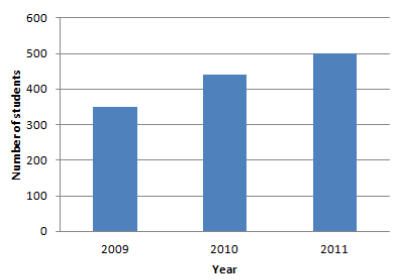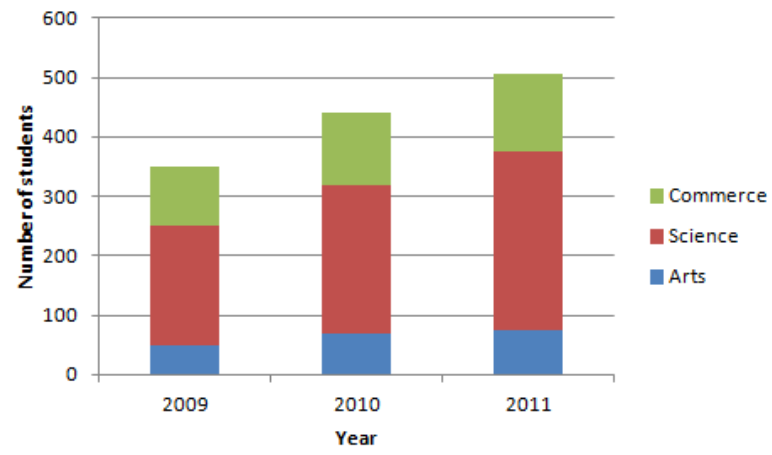Courses
Courses for Kids
Free study material
Free LIVE classes
MoreLIVE
Join Vedantu’s FREE Mastercalss

# What is a subdivided bar diagram? Draw a subdivided bar diagram from the following data?Stream Number of students200920102011Arts507075Science200250300Commerce100120130Verified
321.6k+ views
Hint: First of all, we have to write the definition of a subdivided diagram then we have to draw the subdivided diagram for the above data. To draw the subdivided diagram, we are going to add the number of students in 2009 which are from all three streams. Similarly, we are going to add the students in 2010 and 2011. Now, while drawing the bar diagram, we put the number of students of different streams on the vertical axis and year on the horizontal axis. Using this information, we will draw the subdivided bar diagram.

Complete step-by-step solution:
A subdivided bar diagram is a way of representing data in which the total magnitude is divided into different segments. In this diagram, first of all, we draw the simple bars for each class taking the total magnitude in that class then we divide that bar into segments of its various components.
In the above problem, we have given the following table through which we have to draw a subdivided diagram:
 Stream Number of students 2009 2010 2011 Arts 50 70 75 Science 200 250 300 Commerce 100 120 130

Now, to draw a subdivided bar diagram, first of all, we are going to add all the students in each year from 2009 to 2011. And we are tabulating this total number in the above table as follows:
 Stream Number of students 2009 2010 2011 Arts 50 70 75 Science 200 250 300 Commerce 100 120 130 Total students 350 440 500

To draw a bar diagram, we place years on the horizontal axis and the number of students on the vertical axis. Then we mark 2009, 2010, and 2011 on the horizontal axis. After that, we draw a bar corresponding to the total number of students in that particular year.After that, we divide each bar into segments and that segment corresponds to the number of students in arts, science, and commerce streams.From the above diagram, you can see that we have divided 350 students in 2009 into 50 students in arts, 200 students in science, and 100 students in commerce. The blue color bar is for arts stream, red color for science, and green for commerce.
Hence, we have drawn the subdivided bar diagram.

Note: You might think that instead of adding the students of all streams in a year, we can directly draw the bars of different streams in a year like in 2009, first, we draw a bar for art students then above that we draw a bar for science students and above that, we draw a bar for commerce students. You can do it but it's better if you total the students and then make the segments as it will reduce your calculation mistakes also. Like, instead of drawing a bar for 100 students in commerce you might have drawn a bar for say 150 students in commerce then if you would have first drawn the bar for total students then make the segments you will quickly recognize that you have drawn the wrong bar which you cannot check if you have not drawn the total students bar.

Last updated date: 04th Oct 2023
Total views: 321.6k
Views today: 9.21k